# Insertion Sort In Java – Insertion Sort Algorithm & Examples

This Tutorial Explains Insertion Sort in Java Including its Algorithm, Pseudo-code, and Examples of Sorting Arrays, Singly Linked and Doubly Linked List:

The Insertion Sort Algorithm technique is similar to Bubble sort but, is slightly more efficient. Insertion sort is more feasible and effective when a small number of elements is involved. When the data set is larger, it will take more time to sort the data.

What You Will Learn: [hide]

• Introduction To Insertion Sort In Java
• Insertion Sort Algorithm
• Pseudocode For Insertion Sort
• Sorting An Array Using Insertion Sort
• Insertion Sort Implementation In Java
• Sorting A Linked List Using Insertion Sort
• Sorting A Doubly-Linked List Using Insertion Sort
• Frequently Asked Questions
• Conclusion
• Recommended Reading

## Introduction To Insertion Sort In Java

In the Insertion sort technique, we assume that the first element in the list is already sorted and we begin with the second element. The second element is compared with the first and swapped if not in order. This process is repeated for all the subsequent elements.

In general, the Insertion sort technique compares each element with all of its previous elements and sorts the element to place it in its proper position.

As already mentioned, the Insertion sort technique is more feasible for a smaller set of data, and thus arrays with a small number of elements can be sorted using efficiently Insertion sort.

Insertion sort is especially useful in sorting linked list data structures. As you know, Linked lists have pointers pointing to its next element (singly linked list) and previous element (double linked list). This makes it easier to keep track of the previous and next elements.

Thus it is easier to use Insertion sort for sorting linked lists. However, sorting will take a lot of time if the data items are more.

In this tutorial, we will discuss the Insertion sort technique including its algorithm, pseudo-code, and examples. We will also implement Java programs to Sort an array, Singly linked list, and Doubly linked list using Insertion sort.

### Insertion Sort Algorithm

The insertion sort algorithm is as follows.

Step 1: Repeat Steps 2 to 5 for K = 1 to N-1

Step 2: set temp = A[K]

Step 3: set J = K – 1

Step 4:

Repeat while temp <=A[J]
set A[J + 1] = A[J]
set J = J – 1
[end of inner loop]

Step 5:

set A[J + 1] = temp
[end of loop]

Step 6: exit

As you know, insertion sort starts from the second element assuming that the first element is already sorted. The above steps are repeated for all the elements in the list from the second element onwards and put in their desired positions.

### Pseudocode For Insertion Sort

The pseudo-code for the insertion sort technique is given below.

```procedure insertionSort(array,N )
array – array to be sorted
N- number of elements
begin
int freePosition
int insert_val

for i = 1 to N -1 do:

insert_val = array[i]
freePosition = i

//locate free position to insert the element
while freePosition > 0 and array[freePosition -1] > insert_val do:
array [freePosition] = array [freePosition -1]
freePosition = freePosition -1
end while
//insert the number at free position
array [freePosition] = insert_val
end for
end procedure```

Next, let us see an illustration that demonstrates sorting an array using Insertion sort.

### Sorting An Array Using Insertion Sort

Let us take an example of Insertion sort using an array.

The array to be sorted is as follows: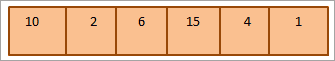Now for each pass, we compare the current element to all its previous elements. So in the first pass, we start with the second element.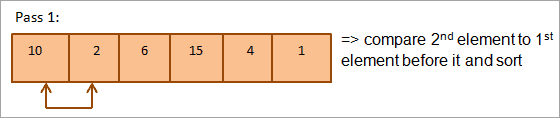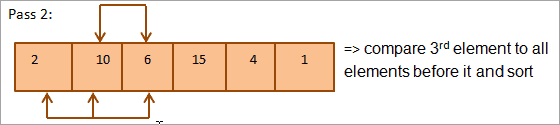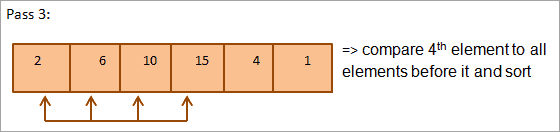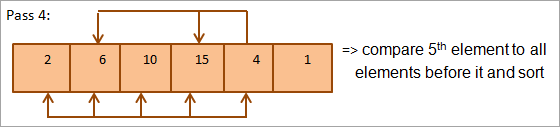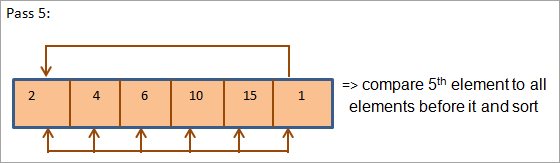Thus, we require N number of passes to completely sort an array containing N number of elements.

The above illustration can be summarized in tabular form as shown below:

PassUnsorted listcomparisonSorted list
1{10,2,6,15,4,1}{10,2}{2,10, 6,15,4,1}
2{2,10, 6,15,4,1}{2,10, 6}{2,6, 10,15,4,1}
3{2,6, 10,15,4,1}{2,6, 10,15}{2,6, 10,15,4,1}
4{2,6, 10,15,4,1}{2,6, 10,15,4}{2,4,6, 10,15,1}
5{2,4,6, 10,15,1}{2,4,6, 10,15,1}{1,2,4,6, 10,15}
6{}{}{1,2,4,6, 10,15}

As shown in the illustration above, at the end of each pass, one element goes in its proper place. Hence in general, to place N elements in their proper place, we need N-1 passes.

### Insertion Sort Implementation In Java

The following program shows the implementation of the Insertion sort in Java. Here, we have an array to be sorted using the Insertion sort.

 `import` `java.util.*;``public` `class` `Main {  ``public` `static` `void` `main(String[] args) {  ``    ``//declare an array and print the original contents``    ``int``[] numArray = {``10``,``6``,``15``,``4``,``1``,``45``};  ``    ``System.out.println(``"Original Array:"` `+ Arrays.toString(numArray));``    ``//apply insertion sort algorithm on the array``    ``for``(``int` `k=``1``; k=``0` `&& temp <= numArray[j])   {  ``            ``numArray[j+``1``] = numArray[j];   ``            ``j = j-``1``;  ``        ``}  ``        ``numArray[j+``1``] = temp;  ``    ``}  ``    ``//print the sorted array``    ``System.out.println(``"Sorted Array:"` `+ Arrays.toString(numArray));``}  ``}  `

Output:

Original Array:[10, 6, 15, 4, 1, 45]
Sorted Array:[1, 4, 6, 10, 15, 45]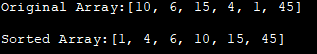In the above implementation, it is seen that sorting begins from the 2nd element of the array (loop variable j = 1) and then the current element is compared to all its previous elements. The element is then placed in its correct position.

Insertion sort works effectively for smaller arrays and for arrays that are partially sorted where the sorting is completed in fewer passes.

Insertion sort is a stable sort i.e. it maintains the order of equal elements in the list.

### Sorting A Linked List Using Insertion Sort

The following Java program shows the sorting of a singly linked list using the Insertion sort.

 `import` `java.util.*;``class` `Linkedlist_sort  { ``    ``node head; ``    ``node sorted; ``    ``//define node of a linked list``    ``class` `node    { ``        ``int` `val; ``        ``node next; ``        ``public` `node(``int` `val)  ``        ``{ ``            ``this``.val = val; ``        ``} ``    ``} ``    ``//add a node to the linked list``    ``void` `add(``int` `val)  { ``        ``//allocate a new node``        ``node newNode = ``new` `node(val); ``        ``//link new node to list``        ``newNode.next = head; ``        ``//head points to new node``        ``head = newNode; ``    ``} ``  ``// sort a singly linked list using insertion sort ``    ``void` `insertion_Sort(node headref)   { ``        ``// initially, no nodes in sorted list so its set to null ``        ``sorted = ``null``; ``        ``node current = headref; ``        ``// traverse the linked list and add sorted node to sorted list``        ``while` `(current != ``null``)  { ``            ``// Store current.next in next``            ``node next = current.next; ``            ``// current node goes in sorted list ``            ``Insert_sorted(current); ``            ``// now next becomes current ``            ``current = next; ``        ``} ``        ``// update head to point to linked list ``        ``head = sorted; ``    ``} ``     ``//insert a new node in sorted list``    ``void` `Insert_sorted(node newNode)   { ``        ``//for head node``        ``if` `(sorted == ``null` `|| sorted.val >= newNode.val)    { ``            ``newNode.next = sorted; ``            ``sorted = newNode; ``        ``} ``        ``else`  `{ ``            ``node current = sorted; ``            ``//find the node and then insert``            ``while` `(current.next != ``null` `&& current.next.val < newNode.val)   { ``                ``current = current.next; ``            ``} ``            ``newNode.next = current.next; ``            ``current.next = newNode; ``        ``} ``    ``} ``  ``    ``//display nodes of the linked list``    ``void` `print_Llist(node head)   { ``        ``while` `(head != ``null``)   { ``            ``System.out.print(head.val + ``" "``); ``            ``head = head.next; ``        ``} ``    ``} ``}``class` `Main{``    ``public` `static` `void` `main(String[] args)  ``    ``{ ``        ``//define linked list object``        ``Linkedlist_sort list = ``new` `Linkedlist_sort(); ``        ``//add nodes to the list``        ``list.add(``10``); ``        ``list.add(``2``); ``        ``list.add(``32``); ``        ``list.add(``8``); ``        ``list.add(``1``); ``        ``//print the original list``        ``System.out.println(``"Original Linked List:"``); ``        ``list.print_Llist(list.head); ``        ``//sort the list using insertion sort``        ``list.insertion_Sort(list.head); ``        ``//print the sorted list``        ``System.out.println(``"\nSorted Linked List:"``); ``        ``list.print_Llist(list.head); ``    ``} ``}`

Output:

Original Linked List:
1 8 32 2 10
Sorted Linked List:
1 2 8 10 32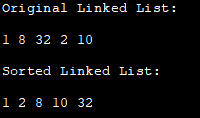In the above program, we have defined a class that creates a linked list and adds nodes to it as well as sorts it. As the singly linked list has a next pointer, it is easier to keep a track of nodes when sorting the list.

### Sorting A Doubly-Linked List Using Insertion Sort

The following program sorts a doubly-linked list using Insertion sort. Note that as the doubly linked list has both previous and next pointers, it is easy to update and relink the pointers while sorting the data.

 `class` `Main {``// doubly linked list node ``static` `class` `Node  {  ``    ``int` `data;  ``    ``Node prev, next;  ``};  ``// return a new node in DLL``static` `Node getNode(``int` `data){  ``    ``//create new node``    ``Node newNode = ``new` `Node(); ``    ``// assign data to node  ``    ``newNode.data = data;  ``    ``newNode.prev = newNode.next = ``null``;  ``    ``return` `newNode;  ``}  ``          ``// insert a node in sorted DLL ``static` `Node insert_Sorted(Node head_ref, Node newNode)  {  ``    ``Node current;  ``      ``//list is empty  ``    ``if` `(head_ref == ``null``)  ``        ``head_ref = newNode;  ``      ``// node is inserted at the beginning of the DLL  ``    ``else` `if` `((head_ref).data >= newNode.data)     {  ``        ``newNode.next = head_ref;  ``        ``newNode.next.prev = newNode;  ``        ``head_ref = newNode;  ``    ``}  ``    ``else`   `{  ``        ``current = head_ref;  ``  ``        ``// find the node after which new node is to be inserted  ``        ``while` `(current.next != ``null` `&&  ``            ``current.next.data < newNode.data)  ``            ``current = current.next;  ``          ``//update the pointers``        ``newNode.next = current.next;  ``          ``if` `(current.next != ``null``)  ``            ``newNode.next.prev = newNode;  ``    ``current.next = newNode;  ``        ``newNode.prev = current;  ``    ``}  ``    ``return` `head_ref; ``}  ``// sort a doubly linked list using insertion sort  ``static` `Node insertion_Sort(Node head_ref) {  ``    ``// sorted doubly linked list - initially empty ``    ``Node sorted = ``null``;  ``      ``// Traverse the DLL and insert nodes to sorted list``    ``Node current = head_ref;  ``    ``while` `(current != ``null``) {``        ``// current.next goes into next  ``        ``Node next = current.next;  ``          ``// set all links to null  ``        ``current.prev = current.next = ``null``;  ``          ``// current goes in sorted DLL  ``        ``sorted=insert_Sorted(sorted, current);  ``          ``// next now becomes current  ``        ``current = next;  ``    ``}  `` ``// Update head_ref to point to sorted doubly linked list  ``    ``head_ref = sorted; ``    ``return` `head_ref; ``}  `` ``// function to print the doubly linked list  ``static` `void` `print_DLL(Node head)  {  ``    ``while` `(head != ``null``)   {  ``        ``System.out.print(head.data + ``" "``);  ``        ``head = head.next;  ``    ``}  ``}  ``  ``// add new node to DLL at the beginning ``static` `Node addNode(Node head_ref, ``int` `newData){  ``    ``// create a new node ``    ``Node newNode = ``new` `Node();  ``      ``// assign data``    ``newNode.data = newData;  ``      ``// Make next of new node as head and previous as null  ``    ``newNode.next = (head_ref);  ``    ``newNode.prev = ``null``;  ``// head=>prev points to new node / ``    ``if` `((head_ref) != ``null``)  ``        ``(head_ref).prev = newNode;  ``      ``// move the head to point to the new node / ``    ``(head_ref) = newNode; ``      ``    ``return` `head_ref; ``}  ``public` `static` `void` `main(String args[]) {  ``    ``// create empty DLL ``    ``Node head = ``null``;  ``  ``    ``// add nodes to the DLL  ``    ``head=addNode(head, ``5``);  ``    ``head=addNode(head, ``3``);  ``    ``head=addNode(head, ``7``);  ``    ``head=addNode(head, ``2``);  ``    ``head=addNode(head, ``11``);  ``    ``head=addNode(head, ``1``);  ``  ``    ``System.out.println( ``"Original doubly linked list:"``);  ``    ``print_DLL(head);  ``  ``    ``head=insertion_Sort(head);  ``  ``    ``System.out.println(``"\nSorted Doubly Linked List:"``);  ``    ``print_DLL(head);  ``  ``}  ``}`

Output:

Original doubly linked list:
1 11 2 7 3 5
Sorted Doubly Linked List:
1 2 3 5 7 11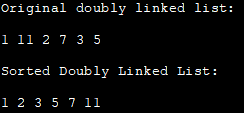### Frequently Asked Questions

Q #1) What is Insertion Sort in Java?

Answer: Insertion sort is a simple sorting technique in Java that is efficient for a smaller data set and in place. It is assumed that the first element is always sorted and then each subsequent element is compared to all its previous elements and placed in its proper position.

Q #2) Why is Insertion Sort better?

Answer: Insertion sort is faster for smaller data sets when the other techniques like quick sort add overhead through recursive calls. Insertion sort is comparatively more stable than the other sorting algorithms and requires less memory. Insertion sort also works more efficiently when the array is almost sorted.

Q #3) What is the Insertion Sort used for?

Answer: Insertion sort is mostly used in computer applications that build complex programs like file searching, path-finding, and data compression.

Q #4) What is the efficiency of Insertion Sort?

Answer: Insertion sort has an Average case performance of O (n^2). The best case for insertion sort is when the array is already sorted and it is O (n). Worst-case performance for insertion sort is again O (n^2).

## Conclusion

Insertion sort is a simple sorting technique that works on Arrays or linked lists. It is useful when the data set is smaller. As the data set gets bigger, this technique becomes slower and inefficient.

The Insertion sort is also more stable and in-place than the other sorting techniques. There is no memory overhead as no separate structure is used for storing sorted elements.

Addition sort functions admirably on arranging connected records that are both separately and doubly-connected records. This is on the grounds that the connected rundown is comprised of hubs that are associated through pointers. Henceforth arranging of hubs gets simpler.